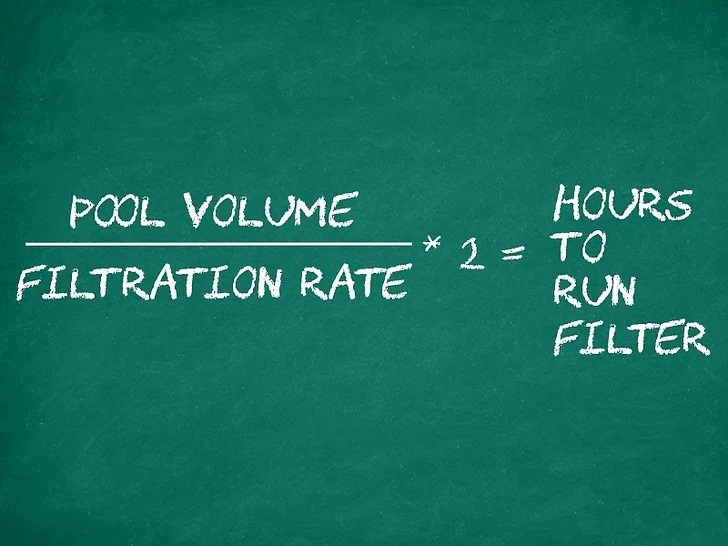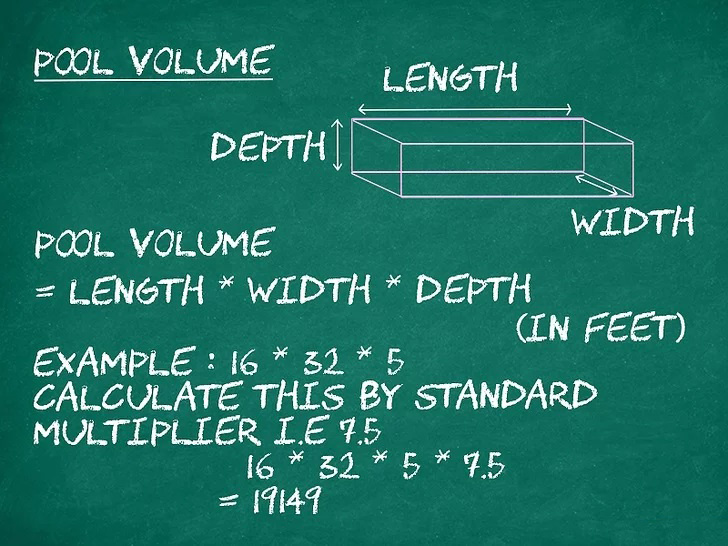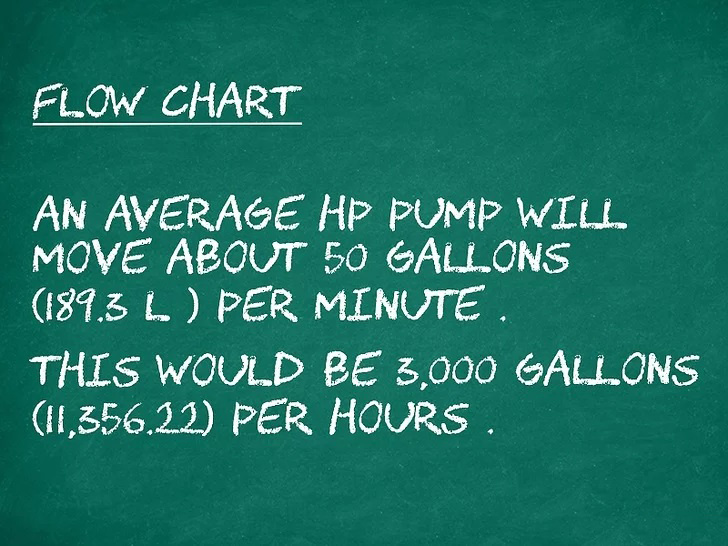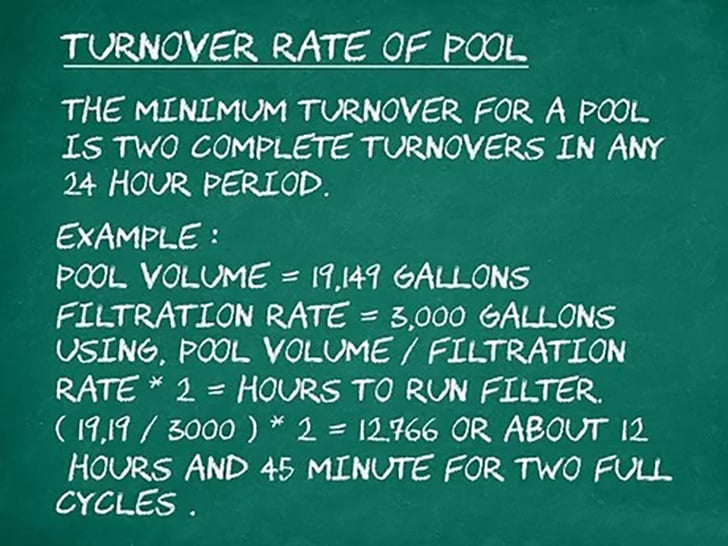# Swimming pool filtration

• Posted on
• 0### A pool is a complete system that needs maintenance to keep your concrete swimming pool crystal clear and fresh. Water clarity is a combination of maintaining the chemical balance of your pool and proper filtration.Use the following equation:

(Pool volume ÷ Filtration level) x 2 = Hours to run the filter.

This tells you how many hours you need to run the pump to fully filter the water 2 times.Determine the volume of your pool. How long you run the filter depends on the size of the pool in relation to the filter.

- If you have a small filter, you'll need to run it longer, depending on how many litres it can handle in a given time.

- Calculate the volume of your pool by multiplying its length x its width x its average depth in metres.

- Multiply this by a standard value. This is 1000 for rectangular and square pools, 890 for an oval and 780 for a circular pool.
For example: 5*10*1.5*1000= 75,000 litres. This gives you the volume of the pool in litres for this 5 x 10 pool with an average depth of 1.5 metres.Determine the flow rate (the amount of water flowing through) of the pump.

Do not forget to include the resistance to flow in your water pipe.

- The pump manufacturer can tell you which flow rate goes with which resistance.

- You can estimate the resistance of your pool water pipework to be 12m/kg for small pools and 24ft/kg for larger pools, or in installations where the pool pump is far away from the pool itself.

- Waterfalls and raised solar panels will increase the resistance of the pipe.

- An average 1 HP pump will move approximately 190 litres per minute. This is approximately 11,400 litres per hour.Calculate the full flow time of your pool.

The minimum recommended flow time for a pool is twice the total volume in 24 hours.

For example: with a pool volume of 75,000 litres and a filtration level of 11,000 litres per hour:

- (Pool volume ÷ Filtration level) x 2 = Hour that the filter should run

- (75,000 ÷ 11,400) x 2 = 13.157, or approximately 13 hours and 10 minutes for two full cycles.

CRISTAL BLUE

"more swimming through technique"